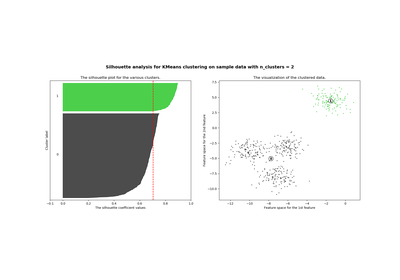# `sklearn.metrics`.silhouette_samples¶

`sklearn.metrics.``silhouette_samples`(X, labels, metric=’euclidean’, **kwds)[source]

Compute the Silhouette Coefficient for each sample.

The Silhouette Coefficient is a measure of how well samples are clustered with samples that are similar to themselves. Clustering models with a high Silhouette Coefficient are said to be dense, where samples in the same cluster are similar to each other, and well separated, where samples in different clusters are not very similar to each other.

The Silhouette Coefficient is calculated using the mean intra-cluster distance (`a`) and the mean nearest-cluster distance (`b`) for each sample. The Silhouette Coefficient for a sample is ```(b - a) / max(a, b)```. Note that Silhouette Coefficient is only defined if number of labels is 2 <= n_labels <= n_samples - 1.

This function returns the Silhouette Coefficient for each sample.

The best value is 1 and the worst value is -1. Values near 0 indicate overlapping clusters.

Read more in the User Guide.

Parameters: X : array [n_samples_a, n_samples_a] if metric == “precomputed”, or, [n_samples_a, n_features] otherwise Array of pairwise distances between samples, or a feature array. labels : array, shape = [n_samples] label values for each sample metric : string, or callable The metric to use when calculating distance between instances in a feature array. If metric is a string, it must be one of the options allowed by `sklearn.metrics.pairwise.pairwise_distances`. If X is the distance array itself, use “precomputed” as the metric. **kwds : optional keyword parameters Any further parameters are passed directly to the distance function. If using a `scipy.spatial.distance` metric, the parameters are still metric dependent. See the scipy docs for usage examples. silhouette : array, shape = [n_samples] Silhouette Coefficient for each samples.

References

## Examples using `sklearn.metrics.silhouette_samples`¶Selecting the number of clusters with silhouette analysis on KMeans clustering